Electricity and Magnetism

# Series and parallel capacitors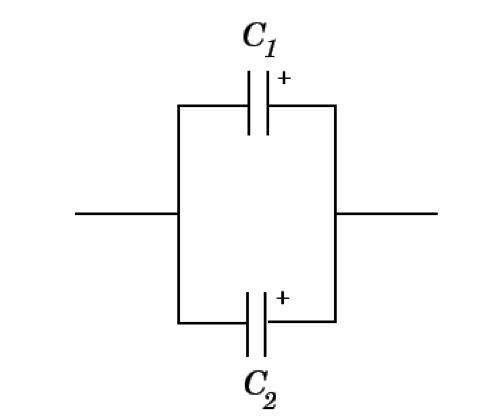Given $C_1 = 5.0 \times 10^{-12} \text{ F}$ and $C_2 = 9.0 \times 10^{-12} \text{ F},$ what is the equivalent capacitance of the circuit above?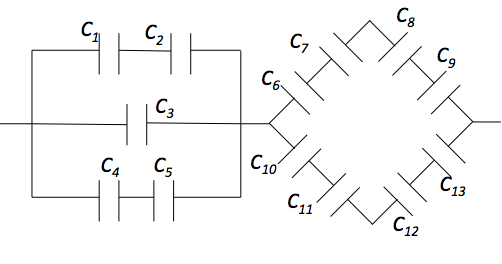Given $C_1 = 5.0 \text{ F},$ $C_2 = 9.0 \text{ F},$ $C_3 = 9.0 \text{ F},$ $C_4 = 6.0 \text{ F},$ $C_5 = 8.0 \text{ F},$ $C_6 = C_7 =C_8 = C_9 = 32.0 \text{ F},$ and $C_{10} = C_{11} = C_{12} = C_{13} = 24.0 \text{ F},$ what is the approximate overall equivalent capacitance of the circuit above?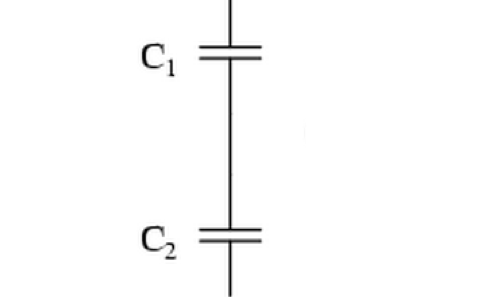Given $C_1 = C_2=4 \text{ F},$ what is the equivalent capacitance of the two capacitors?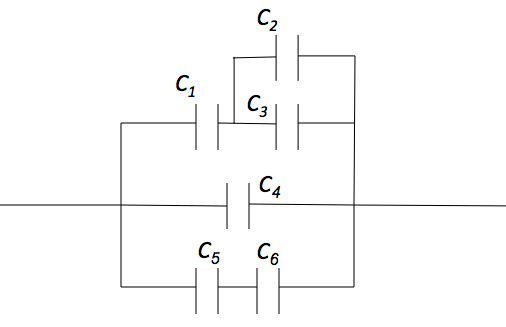Find the approximate equivalent capacitance of the combination of capacitors in the above figure, where $C_1 = 5.0 \text{ F},$ $C_2 = 5.0 \text{ F}.$ $C_3 = 5.0 \text{ F},$ $C_4 = 10.0 \text{ F},$ $C_5 = 10.0 \text{ F}$ and $C_6 = 10.0 \text{ F}.$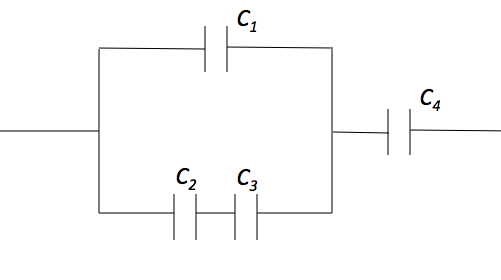Given $C_1 = 8.0 \text{ F},$ $C_2 = 7.0 \text{ F},$ $C_3 = 6.0 \text{ F} ,$ and $C_4 = 6.0 \text{ F},$ what is the approximate overall equivalent capacitance of the circuit above?

×